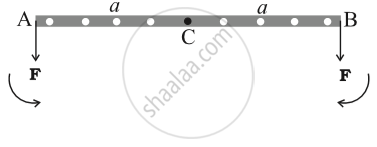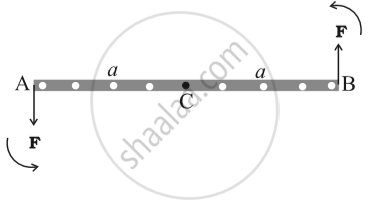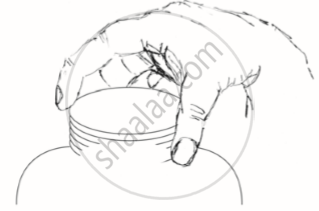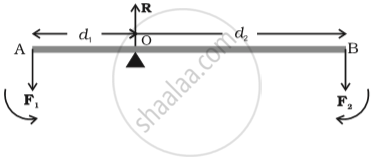ISC (Commerce) Class 11CISCE
Share

# Equilibrium of Rigid Bodies

#### description

Principle of moments

• Centre of gravity

### Equilibrium of Rigid Body

• A force changes the translational state of the motion of the rigid body, i.e. it changes its total linear momentum.

• A torque changes the rotational state of motion of the rigid body, i.e. it changes the total angular momentum of the body

Note: Unless stated otherwise, we shall deal with only external forces and torques.

A rigid body is said to be in mechanical equilibrium, if both its linear momentum and angular momentum are not changing with time. This means

Total torque should be zero => Rotational EquilibriumFnet = 2F
τnet = 0

• Total force should be zero => Translational EquilibriumFnet = 0
τnet = Fl

A pair of equal and opposite forces with different lines of action is known as a couple or torque. A couple produces rotation without translation.

When you open the lid of a jar, you apply couple on it.An ideal lever is essentially a light rod pivoted at a point along its length. This point is called the fulcrum

The lever is a system in mechanical equilibrium.Mechanical advantage = "F1"/"F2" = "d1"/"d2"

Mechanical advantage greater than one means that a small effort can be used to lift a large load.

Centre of Gravity

• The centre of gravity of a body is that point where the total gravitational torque on the body is zero.

• The centre of gravity of the body coincides with the centre of mass in uniform gravity or gravity-free space.

• If g varies from part to part of the body, then the centre of gravity and centre of mass will not coincide.

### Shaalaa.com

Rotational equilibrium [01:10:45]
S
0%

S